[BACK]
 Computers, Materials & ContinuaDOI:10.32604/cmc.2022.027830Article

Application of the Fictitious Domain Method for Navier-Stokes Equations

1Al-Farabi Kazakh National University, Almaty, Kazakhstan
2Abai Kazakh National Pedagogical University, Almaty, Kazakhstan
3Sarsen Amanzholov East Kazakhstan University, Ust-Kamenogorsk, Kazakhstan
*Corresponding Author: Zhadra Zhaksylykova. Email: zhako83@mail.ru
Received: 26 January 2022; Accepted: 02 April 2022

Abstract: To apply the fictitious domain method and conduct numerical experiments, a boundary value problem for an ordinary differential equation is considered. The results of numerical calculations for different values of the iterative parameter τ and the small parameter ε are presented. A study of the auxiliary problem of the fictitious domain method for Navier-Stokes equations with continuation into a fictitious subdomain by higher coefficients with a small parameter is carried out. A generalized solution of the auxiliary problem of the fictitious domain method with continuation by higher coefficients with a small parameter is determined. After all the above mathematical studies, a computational algorithm has been developed for the numerical solution of the problem. Two methods were used to solve the problem numerically. The first variant is the fictitious domain method associated with the modification of nonlinear terms in a fictitious subdomain. The model problem shows the effectiveness of using such a modification. The proposed version of the method is used to solve two problems at once that arise while numerically solving systems of Navier-Stokes equations: the problem of a curved boundary of an arbitrary domain and the problem of absence of a boundary condition for pressure in physical formulation of the internal flow problem. The main advantage of this method is its universality in development of computer programs. The second method used calculation on a uniform grid inside the area. When numerically implementing the solution on a uniform grid inside the domain, using this method it’s possible to accurately take into account the boundaries of the curved domain and ensure the accuracy of the value of the function at the boundaries of the domain. Methodical calculations were carried out, the results of numerical calculations were obtained. When conducting numerical experiments in both cases, quantitative and qualitative indicators of numerical results coincide.

Keywords: Fictitious domain method; Navier-Stokes equations; difference schemes; approximation; computational algorithm; numerical experiment

1  Introduction

At the beginnig we will give a literature review on the application of the fictitious domain method. Currently, there are several methods for the numerical solution of boundary value problems in complex geometric domains, such as the method of curved grids and the fictitious domain method. The construction of curved grids for the numerical solution of problems requires the transformation of the equation into curved coordinates, which has a more complex form than the original equations. When constructing curved grids, various requirements are imposed on difference equations, which makes the construction of curved grids a difficult mathematical task. Therefore, for the numerical solution of a wide class of problems of mathematical physics in an arbitrary domain, it is effective to use the fictitious domain method [1,2]. The fundamental works on the application of the fictitious domain method include the works of Vabischevich et al. . Works [1,2] are devoted to the fictitious domain method in the numerical solution of problems of mathematical physics in complex areas. The fictitious domain method is based on the transition to a problem in a regular area that entirely contains the original one. The issues of substantiation of such an approach at the differential level in the study of boundary value problems for elliptic and parabolic equations, eigenvalue problems are considered. Modifications of well-known iterative methods are constructed to solve grid problems that arise when using the fictitious domain method. The possibilities of the fictitious domain method are illustrated by examples of solving problems of ideal and viscous incompressible fluid, filtration under a hydraulic structure. In [3,4], the fictitious domain method is applied to elliptic equations. Error estimates are obtained for solving problems using the fictitious domain method. Lagrange multipliers based on methods of fictitious domains are applied. Papers  are devoted to the application of the fictitious domain method for solving computational fluid dynamics problems.

The fictitious domain method for the Navier-Stokes equations is the subject of works by Bugrov et al. . Smagulov et al.  proposed the fictitious domain method for hydrodynamic equations in multiply connected domains.

In , a variation of the fictitious domain method for the Navier-Stokes equations for a viscous incompressible fluid in the velocity and pressure variables was proposed. Moreover, a constant value was imposed on pressure at the boundary of the auxiliary domain. This condition allows obtaining the Dirichlet problem for the Poisson equation for pressure which admits the development and application of effective iterative numerical methods for its solution.

The fictitious domain method is utilized in solving problems of computational fluid dynamics such as modeling the motion of particles in a fluid flow , the motion of an incompressible fluid , the two-phase Stokes problem including the surface tension force , the problem of flow around moving or deformable bodies fluid flow  and others.

In , the spectral element/HP method was applied to perform direct numerical simulation (DNS) for one row of turbine blades T106A using open source software Nektar++. The main goal of the current research is to perform preliminary researches for uniform, stable flow around an aerodynamic profile using the Nektar++ solver for 2D Navier-Stokes equations for incompressible flow.

In , the fictitious domain method was applied to simulate the interaction of liquid particles with the Navier slip boundary condition. Numerical experiments show that the method works well for an anisotropic particle in a flow with the Navier slip boundary condition.

In , fictitious domain method with a H1 -penalty for the Stokes problem with a Dirichlet boundary condition is studied. Work  is devoted to the application of the fictitious domain method in the numerical simulation of a pulse oscillation converter. In , fictitious domain method with a distributed Lagrange multiplier was studied for parabolic problems of a jump-like type with moving boundaries.

In , the influence of rotation and hydrostatic initial stresses under the influence of an electromagnetic field incident on the outer surface of a semiconductor medium was investigated.

Papers  are devoted to the study of fictitious domain method for problems with discontinuous coefficients. Elliptic type equations with strongly varying coefficients are considered and investigated in . Equations of this type are obtained using fictitious domain method. A special method is used for the numerical solution of an elliptic equation with strongly varying coefficients. For the obtained problem, the convergence rate estimation theorem of the developed numerical algorithm is proved. Based on obtained estimates, a computational algorithm was developed and numerical calculations were performed to illustrate the effectiveness of the proposed method. Work  is devoted to the development of new exact and numerical solutions for the 1-dimensional integro-differential Ito equation using the methods of 1-expansion and finite differences, respectively. Trigonometric, hyperbolic and rational solutions are successfully presented. The stability and accuracy of the obtained numerical simulation are discussed. The presented graphical comparison shows that the exact and numerical solutions almost coincide with each other. Analytical and numerical solutions of the generalized Benjamin-Bona-Mahoney equation (GBBM) are investigated in . The exact solution is obtained analytically, while numerical solutions are demonstrated using some methods, namely adaptive moving mesh and uniform mesh methods. The exact solution is presented in the form of convergent power series. Finite differences are also used to discretize the BBM equation.

The works  are devoted to the application of a parallel computational algorithm for fictitious domain method. In , a parallel computational algorithm is constructed using the fictitious domain method for the three-dimensional Helmholtz equation. Work  is devoted to modeling turbulent flow in a channel using fictitious domain method. The paper  describes the possibilities of using the fictitious domain method for biomechanics problems.

The work  is aimed at studying the effect of rotation on the general model of generalized thermo-microstriction equations for a homogeneous isotropic elastic semi-spatial solid whose surface is subjected to thermal shock. Comparisons are made with the results in the presence and absence of rotation, as well as in the presence and absence of microextension constants between the two theories.

In , the influence of variable thermal conductivity, which depends on temperature, is considered in the context of photothermal diffusion (PTD). The PTD process is applied using the theory of thermoelasticity under chemical action. The presented model describes the interaction between elastic-thermal-plasma waves based on the properties of the material of a semiconductor elastic medium. The Laplace transform is used to solve control equations in one dimension of a thin circular plate. Complete solutions in the time domain are observed using the numerical approximation method. Physical fields with some comparisons are presented analytically and graphically.

The paper  presents the results of a study of the Navier-Stokes equations in numerical modeling in two-connected domains. Two methods are used to solve this equation numerically. In the first method, the condition of unambiguity of pressure is used for numerical solutions of difference equations for the functions of current and velocity vortex. To obtain a numerical solution of the elliptic equation, which is obtained for current functions is found as the sum of two problems. The second alternative method for solving the difference problem, fictitious domain method is considered. In this method, there is no need to satisfy the condition of one-digit pressure, so it is easy to implement.

In , the augmented domain method is considered for numerical simulation of the flow of a viscous incompressible fluid in complex geometric domains. The problem is considered in a discretely given two-connected domain with a curved boundary. Spline interpolation of curved boundaries is performed. A monotonic finite difference scheme and an algorithm for numerical realization of the Navier-Stokes equation for a viscous incompressible fluid are developed. Numerical results are obtained for different numbers of grid nodes.

Glowinski et al. [5,3739] considers a family of domain decomposition methods based on the explicit use of the Lagrange multiplier defined on the actual boundary. The proposed technique associated with true boundary conditions is common for modeling inviscid incompressible potential flows. According to the proposed method, the original differential problem is reduced to an optimal control problem with a saddle point, and the iterative method of conjugate gradients is used for its numerical implementation.

In , the fictitious domain method without Lagrange multipliers is used for numerical simulation of the suspension concentration. The boundary is tracked to delimit the domains occupied by liquid and solid particles. The particle indicator function is constructed using the Heaviside function. Further, the Heaviside function is approximated using the hyperbolic tangent in a small neighborhood (proportional to the grid step) around the boundary. Such a continuous function ensures smooth change, improves numerical accuracy and reliability.

Let us dwell on the advantages and disadvantages of the existing variants of the fictitious domain methods. The method proposed and implemented in [5,3739] allows satisfying the boundary conditions on the actual boundary using variational principles. Two meshes are constructed for the finite element solution in the extended domain: triangulation over the entire domain and a curvilinear mesh on the actual boundary. Then, the desired variables are matched on the curvilinear boundary at each iteration of the conjugate gradient method. In some cases, this process may behave worse than the solution of the original problem on a non-uniform mesh consistent with a curvilinear boundary. The results of numerical calculations show convergence to the solution “in the average” due to the use of variational principles since the functional containing the main equation and the boundary condition is minimized.

Therefore, in this paper, the authors propose a method that allows constructing a homogeneous difference scheme in the entire extended domain which is a convenient tool in terms of programming automation. At the same time, a reasonable continuation of the main equation coefficients leads to the convergence of the solution to the desired solution in the original domain, which is confirmed by mathematically proven statements and the results of numerical calculations.

In this work, two methods are applied for the numerical solution of the formulated problem. The first one is the fictitious domain method associated with the modification of nonlinear terms in a fictitious subdomain. The model problem shows the efficiency of using such a modification. The proposed variation of the method is used to solve two problems at once that arise in the numerical solution of the Navier-Stokes equations: the problem of the curvilinear boundary of an arbitrary domain and the problem of the absence of a boundary condition for pressure in the physical formulation of the internal flow problem.

The second method used the calculation on a uniform mesh inside the domain. The solution on a uniform mesh inside the domain makes it possible to accurately take into account the boundaries of the curved domain and ensures the accuracy of the function value on the boundaries of the domain in the numerical implementation.

2  One-Dimensional Problem

Consider the boundary value problem for the ordinary differential equation:

y2y=2, y(0)=y(0.5)=0(1)

which has the known exact solution:

y=(1e2x)2(e1)+x.

We apply the fictitious domain method for problem (1) in the form

ddx(a(x)dvx)2d(b(x)v)dx=fε(x), 0<x<1(2)

v(0)=v(1)=0(3)

with the agreement conditions imposed at x=0.5:

[v]x=0.5=[a(x)dvdxb(x)v]x=0.5=0.(4)

The coefficients in (2) are defined as follows:

a(x)={1,0<x<0,51ε2,0,5<x<1,fε(x)={2,0<x<0,50,0,5<x<1(5)

If b(x)={1,0<x<0.51ε,0.5<x<1 then the solution to problem (2)(4) has the form

vε(x)={C1+C3e2x+x,0<x<0.5C2+C4e2εx,0.5<x<1,(6)

where C1=12(e1)2ε(1eε)ε(1eε)(e21)(1+eε)(e1)2,

C3=ε(1eε)ε(1eε)(e21)(1+eε)(e1)212(e1)2

C2=εeεε(1eε)(e+1)(1+eε)(e1), C4=εeεε(1eε)(e+1)(1+eε)(e1)

If b(x)=1,0<x<1 then the solution to problem (2)(4) has the form

vε(x)={C1+C3e2x+x,0<x<0.5C2+C4e2ε2x,0.5<x<1(7)

where C1=e12(e+1)(e+eε2),C3=e12(e+1)(e+eε2),C2=eε22(e+eε2),C4=eε22(e+eε2).

By comparing the solutions of the one-dimensional model problem, we see that in the first case, when 0.5<x<1, the solution vε(x) directly proportional to the small parameter ε. Therefore, it can be concluded that in case of b(x)=1ε, 0.5<x<1 the solution of the approximate problem in a fictitious subdomain converges to the solution of the original problem faster than in case of b(x)=1, 0.5<x<1.

We consider the following non-stationary equation for the numerical implementation of the one-dimensional equation of the fictitious domain method by the establishment method:

dvdt=ddx(a(x)dvx)2vd(b(x)v)dxfε(x)(8)

which has the known exact solution:

v=(1e2x)2(e1)+x.

The coefficients are defined as follows:

a(x)={1,0<x<0,51ε2,0,5<x<1, b(x)={1,0<x<0.51ε,0.5<x<1fε(x)={e2x(2x1)1e1e2xe4x(e1)22x,0<x<0,50,0,5<x<1(9)

Let us construct a non-uniform mesh that thickens in the neighborhood of the actual boundary, x=0.5. Introduce the parameter t, 0<t<1. Let us construct a uniform grid according to t, ti=(i1)/N,i=1,2,,N+1. The mesh in the integration domain is defined using the following formula: xi=4(ti0,5)3+0,5,i=1,2,,N+1.

The steps of a non-uniform mesh thickening near x=0.5 are defined as

hi=xixi1,i=1,2,,N,

i=0,5(hi+1+hi).

Then the corresponding difference scheme on a nonuniform mesh has the form

vin+1vinτ=1i[ai+1/2vi+1n+1vin+1hi+1ai1/2vin+1vi1n+1hi][(vin|vin|)(bi+1vi+1n+1bivin+1)hi+1+(vin+|vin|)(bivin+1bi1vi1n+1)hi]fiε.

Fig. 1 and Tab. 1 show the results of numerical calculations for various values of the iterative parameter τ and small parameter ε. Methodological calculations were carried out using two schemes. The calculation results demonstrate good convergence of the auxiliary problem solution of the fictitious domain method to the solution of the main problem in the original domain. In this case, the maximum deviation is 0,00003472.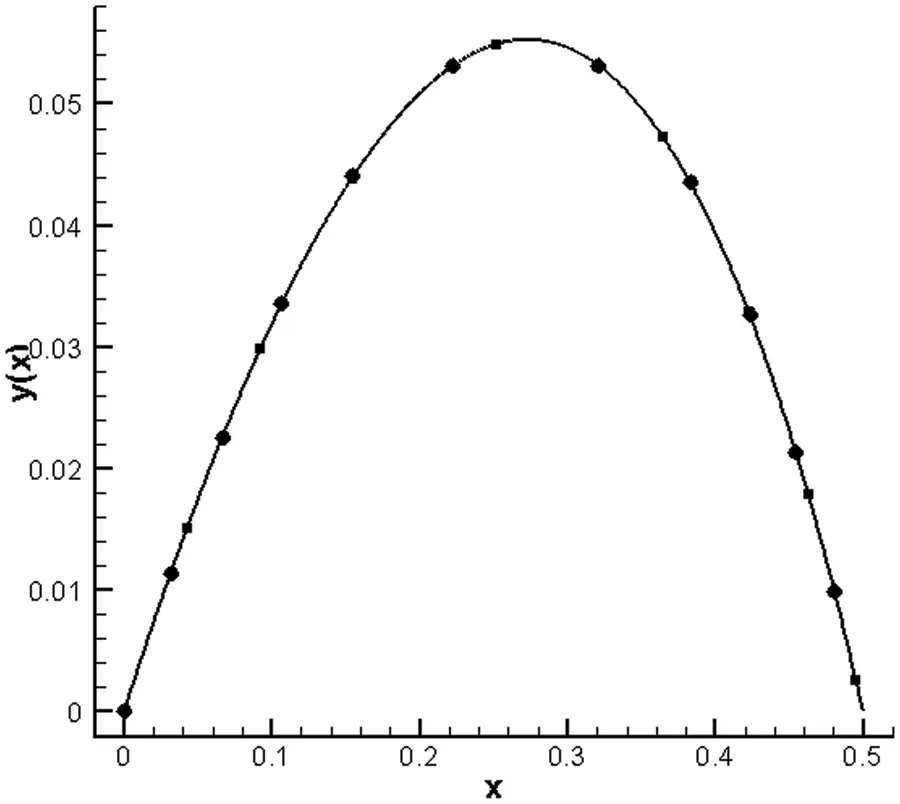Figure 1: Plot of the numerical solution in comparison with the exact solution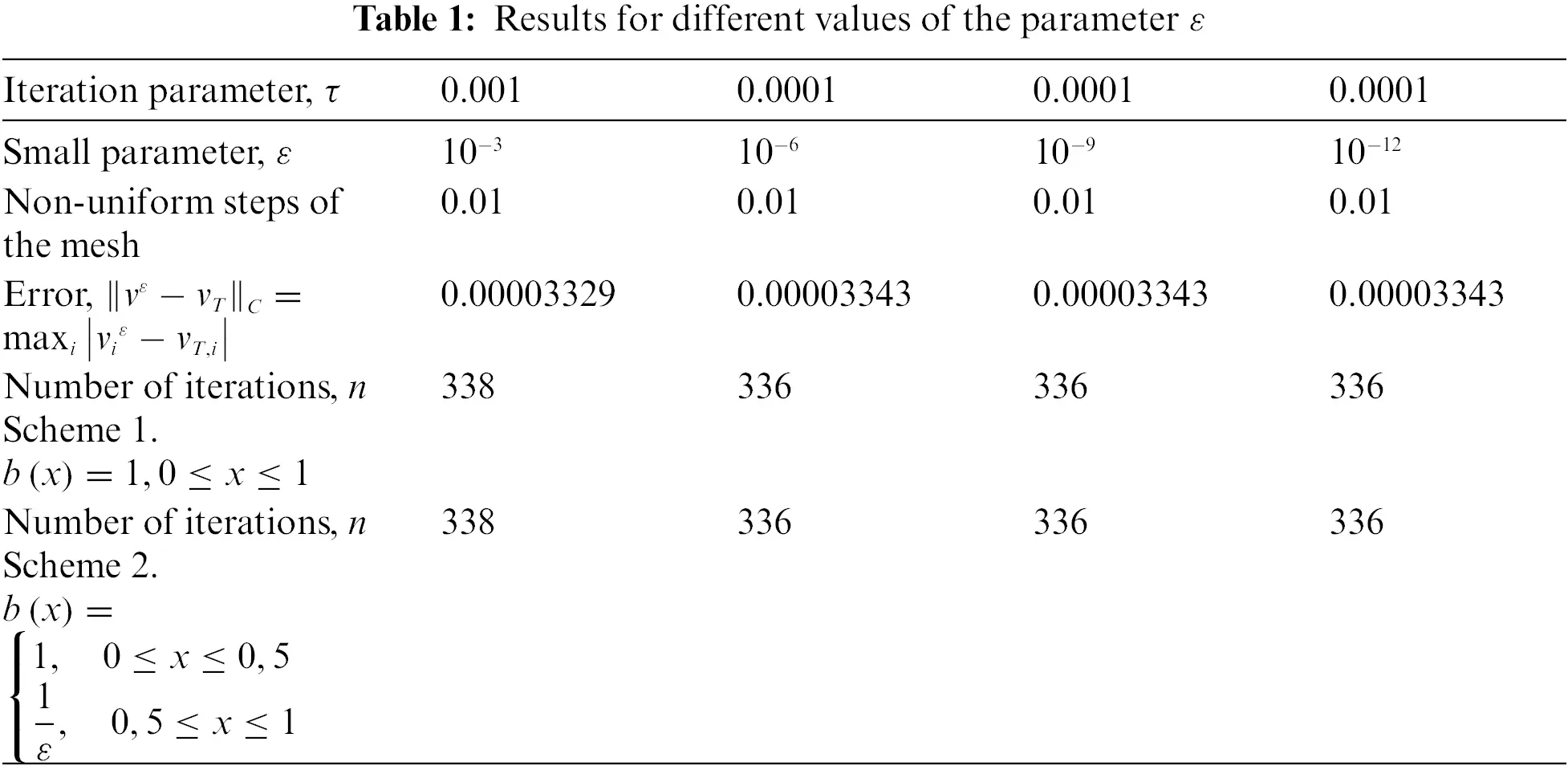3  Multivariable Problem

Formulation of the problem. Let us consider an initial-boundary value problem for an unsteady flow of a viscous incompressible fluid in a bounded domain ΩR2 with a curved border S. The problem is reduced to solving the system of nonlinear Navier-Stokes equations in the velocity-pressure variables:

vt+(v)v=μΔvp+f,(10)

divv=0,(11)

v|t=0=v0(x),v|S=0.(12)

The auxiliary problem corresponding to the fictitious domain method is reduced to solving a system of nonlinear equations with variable coefficients in D=D1Ω with a boundary S is as follows:

vεt+(vε)(αεvε)=div(μεvε)pε+f,(13)

divvε=0,(14)

vε|t=0=0,vετ|S1=0,pε|S1=0(15)

with the agreement condition imposed at the border S:

[(αεvε(δvε)μεvεpεδ)n]|S=0,[vε]|S=0,(16)

where τ is the tangent vector to the boundary S1, [] denotes a jump when passing through S, δ is the metric tensor, n is the normal to the boundary S, and f is continued in D1 with the preservation of the L2(Ω) norm,

με={μ,inΩ,με2,inD1(17)

αε={1,inΩ,1ε,inD(18)

Condition (16) is obtained after a preliminary transformation of the nonlinear terms:

(vε)(aεvε)=(vε)(aεvε)+aεvε(vε)=(aεvε(δvε)).(19)

Let us introduce a set of infinitely differentiable solenoidal in D vector functions v(x) with tangent components vanishing on S1, M(D)={v(x)C(D),divv=0,v(x)τ(x)=0,xS1}. The spaces obtained by closure of M(D) in the L2(D) and Wo21(D) norms are denoted by V(D),V1(D), respectively, and their dual spaces are denoted by V(D) and V1(D), respectively.

Definition 1. The generalized solution to the problem (13)(16) is the function vε belonging to the class L2(0,TV1(D))L(0,TL2(D)) and satisfying the integral identity:

0T(vε,Φt)Ddt0T((vε)Φ,aεvε)Ddt+0TS1(vεΦ)vεndsdt+

+με0TS1k(x)(vΦ)dsdt+0T(μεvεΦ)Ddt=0T(fΦ)Ddt(20)

for any ΦC1(0,TV1(D)),Φ(T)=0,(u,v)D=Duvdx, k(x) is the doubled mean curvature of the boundary S1. Assume that k(x) is a non-negative function.

The following lemma  is used to obtain a priori estimates.

Lemma 1. The following equality holds for a function vε belonging to the class L2(0,TV1(D))L(0,TL2(D)):

(Δvε,Φ)Ω=(vε,Φ)ΩS1k(x)(vε,Φ)dl.(21)

To apply the Galerkin method, consider the eigenvalue problem

Aωj=λjωj,j=1,2,(22)

where

Aωj={μΔωjpj,inΩ,μεΔωjpj,inD1(23)

divωj=0, in D=D1Ω(24)

with the boundary conditions

ωjετ|S1=0,pε|S1=0, j=1,2,,(25)

and the agreement conditions

[μεωnpδn]|S=0,[ωj]|S=0.(26)

The operator A is self-adjoint, and the set of functions {ωj} forms the basis in V1(D). In , these spectral problems were solved numerically.

In matters related to the justification of the existence of solutions to boundary value problems for the Navier-Stokes equations by the Galerkin method, the very fact of the existence of the spectral problem eigenfunctions for the operator (21)(24) is used.

The operator is not symmetric and positive definite in the considered problem (13)(16). Then the Galerkin approximations are as follows:

vNε(t,x)=m=1NαNm(t)ωm(x)(27)

where {ωj(x)}j=1N is the basis of a finite dimensional subspace V1(D).

Assume that L=A+N. Multiply (13) scalarly in V1(D) by an arbitrary function uV1(D) to get the equality

(Av0ε+Nv0εf,u)=0.

Since uV1(D) can be represented as

u=i=1Nbiωi(x),

then αNm(t) is found from the system of ordinary differential equations

ddt(vNε(t),ωj)D+aε((vNε)vNε,ωj)D+μεS1k(x)(vNε,ωj)Dds+

+(μεvNε,ωj)D=(f,ωj)D, j=1,2,,N(28)

vNε(t)|t=0=0,αNm(t)|t=0=0, m=1,2,,N(29)

Taking into account (27), we obtain from (28):

ddt(m=1NαNm(t)ωm(x),ωj)D+aε((m=1NαNm(t)ωm(x))m=1NαNm(t)ωm(x),ωj)D++μεS1k(x)(m=1NαNm(t)ωm(x),ωj)Dds+(μεm=1NαNm(t)ωm(x),ωj(x))D=(f,ωj)D

Further,

dαN,j(t)dt+aε(i=1Nm=1N(αN,iαN,mωi(x)ωm(x)),ωj(x))D+

+μεS1(k(x)ds)αN,j(t)+μεm=1NαNm(t)(ωm(x),ωj(x))D=(f,ωj)D(30)

αN,j(t)|t=0=0, j=1,2,,N(31)

The solvability of systems of Eqs. (30)(31) in time is known from the general theory of ordinary differential equations .

Global solvability follows from a priori estimates of the solution vNε(t,x) which is obtained from the following system:

ddt(vNε(t,x),vNε(t,x))D+aε((vNε)vNε,vNε)D+

+μvNxεL2(Ω)2+μεvNxεL2(D1)2+μεS1k(x)(vNε(t,x))2dsfV1(D)vεV1(D)(32)

By virtue of the continuity equation and boundary conditions, we have

|D((vNε(t,x))vNε(t,x),vNε(t,x))dx|=|S(vNε(t,x))2vNε(t,x)dS|

S1|vNε(t,x)|3dSC0vNε(t,x)L2(D1)2vNε(t,x)L2(D1)(33)

Hence the following inequality is obtained:

12ddtvNε(t,x)L2(D)2+μvNε(t,x)L2(Ω)2+(μεaεC0vNε(t,x)L2(D1))vNε(t,x)L2(D1)2

f(t)V1(D)vNε(t,x)V1(D)2(34)

Let

μεaεC0vNε(t,x)L2(D1)0.(35)

Then

max0tTvNε(t,x)D0Tf(t)V1(D)dt.(36)

Choose ε such that

μεaεC00Tf(t)V1(D)dt0.(37)

Then it follows from inequality (34) that

max0tTvNε(t,x)L2(D)+0TvNε(t,x)L2(Ω)2dt+1ε0TvNε(t,x)L2(D1)2dt+1ε0TS1k(x)|vNε(t,x)|2dsdt C0Tf(t)V1(D)2dtC<

where is the constant C does not depend on ε.

4  Numerical Calculations

We consider the numerical solution of the auxiliary problem (13)(16) to illustrate the advantages of the proposed approach.

Let us take a curvilinear channel with solid boundaries (Fig. 2) as the domain under consideration. D1 denotes the fictitious domain and Ω denotes the physical domain.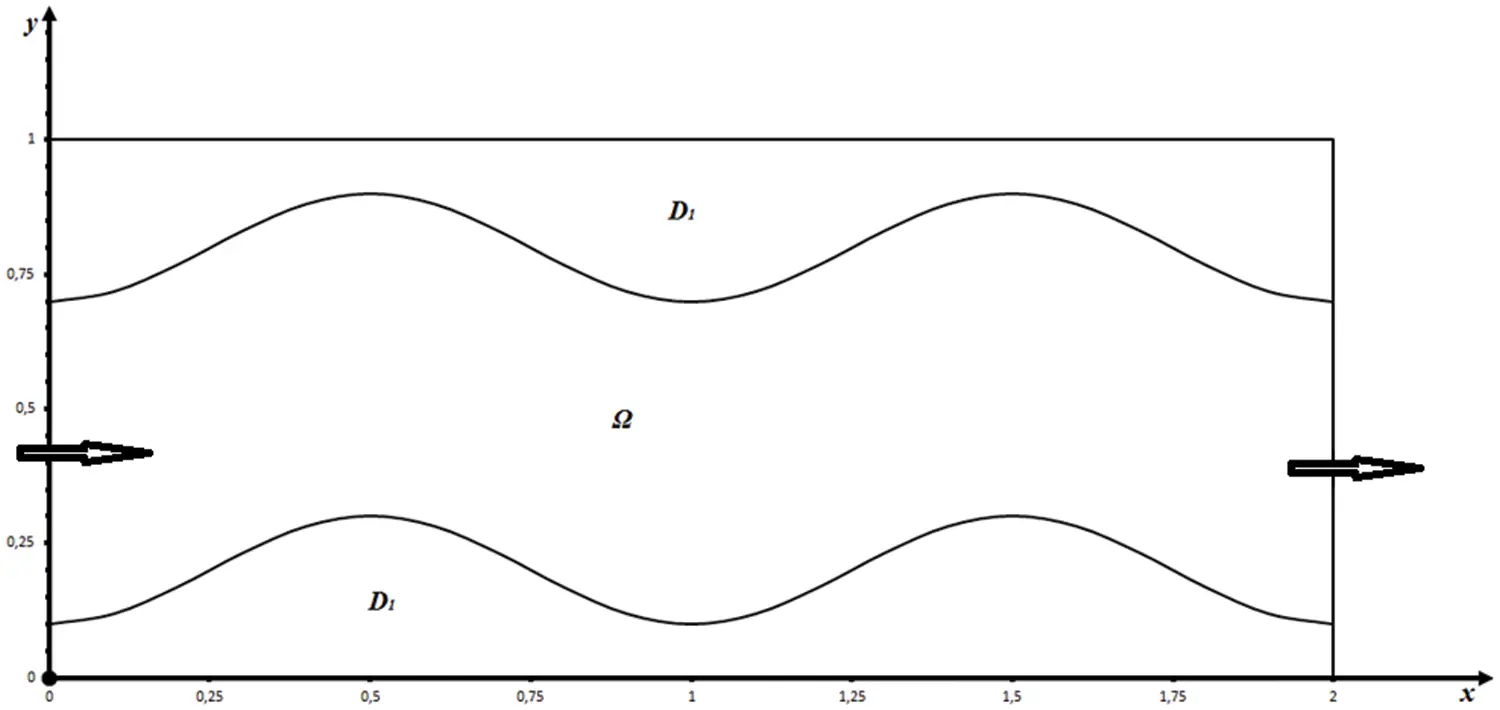Figure 2: Schematic representation of the domain under consideration

We use the finite difference method and the scheme of splitting by physical processes  for the numerical implementation of the auxiliary problem (13)(16). The integration domain is covered by the so-called MAC-grid , in which the nodes for determining the pressure are located inside a rectangular grid cell, and the nodes for determining the velocity component are located on its faces. The scheme taking into account the sign is used when approximating the convective terms. Thus, an approximation with the second order of accuracy in space and the first order in time is provided.

Let the velocity field vn be known at some point in time tn=nτ, where τ is the time step and n is the number of steps. Then the scheme for determining unknown functions at tn+1=(n+1)τ can be represented as a three-stage splitting scheme:

Stage I:

vn+1/2vnτ=(vn)(aεvn+1/2)+μεΔvn+1/2(38)

Stage II:

Δp=vn+1/2τ.(39)

Stage III:

vn+1vn+1/2τ=p,(40)

where με={μ,inΩ,με2,inD1, αε={1,inΩ,1ε,inD1.

The prescribed values of pressure and zero values of the tangential component of the fluid flow velocity at the inlet and outlet of the computational domain were set in the numerical implementation. At the «solid» boundaries, the pressure values are given as linear functions and the tangential component of the velocity is equal to zero.

Obviously, the sum of the equations corresponding to Stages I and III gives the original equation of motion (13), and Eq. (39) corresponding to Stage II is obtained from (40) by applying the divergence operator to the last one taking into account the continuity equation.

The following physical interpretation of the given splitting scheme is proposed. At Stage I, it is assumed that the transfer of momentum (momentum per unit mass) is carried out only due to convection and diffusion. The velocity field thus obtained does not satisfy the incompressibility condition, in general. In this paper, the implicit scheme is used at Stage I in contrast to the classical version of the method of splitting into physical processes . A similar approach was substantiated and numerically implemented in . Iterative schemes were proposed for solving auxiliary grid Navier-Stokes equations. This approach improves the condition number of the system of linear equations matrix and makes it possible to speed up the convergence of the solution.

At Stage II, the pressure field is found from the solution of the Poisson equation based on the found intermediate velocity field and taking into account the solenoidality condition of the velocity vector. At this stage, numerical methods for solving grid elliptic equations with Dirichlet boundary conditions for pressure were used.

At Stage III, it is assumed that the transfer is carried out only due to the pressure gradient, and the convection and diffusion are absent.

In the numerical implementation, grids containing 50×20,100×40,150×60,200×80 nodes and the following dimensionless parameter values were used:

0<xi<2,0<yi<1,τ=0.001,μ=1/Re=0.001,ε=105.

The upper and lower solid curved boundaries are described by the equations

y1=0.20.1cos(2πx)y2=0.80.1cos(2πx)

Currently, there are several methods for the numerical solution of boundary value problems in complex geometric domains, such as the method of curved grids and fictitious domain method. The construction of curved grids for the numerical solution of problems requires the transformation of the equation into curved coordinates, which has a more complex form than the original equations. Therefore, for the numerical solution of a wide class of problems of mathematical physics in an arbitrary domain, it is effective to use the fictitious domain method.

The solution of this problem is implemented in two ways. In the first case, the fictitious domain method by the leading coefficients was used. The main advantage of this method is its versatility in the development of computer programs for the numerical simulation of a wide class of problems of mathematical physics. In the second case, a calculation on a was used. Methodological calculations have been carried out, and the results of numerical calculations have been obtained. The quantitative and qualitative indicators of the numerical results coincide for both cases when conducting numerical experiments.

The boundary of the physical domain is smeared when solving the problem by the fictitious domain method, and therefore the solutions at the boundaries may differ from the boundary condition, although it gives reliable results of the flow. In addition, the fictitious domain method is easily implemented. But since the problem is ill-conditioned at the first stage, an implicit scheme was used where the boundary conditions of the integer iteration step were used in the calculations.

Fig. 3 shows the vector field of the problem solved using the fictitious domain method, respectively, Fig. 4 shows the velocity module of the problem solved using the dummy domain method. Curved lines can be seen from the velocity modulus, Poiseuille currents are formed corresponding to the curvature of the boundary.

Fig. 5 shows the vector field of the problem solved on a consistent grid, respectively, Fig. 6 shows the velocity module of the problem solved on a consistent grid. In Figs. 4 and 6, one can see the qualitative and quantitative correspondences of the formed Poiseuille flows when solving the problem by different methods.

Numerical implementation of the solution on a uniform grid inside the domain makes it possible to accurately take into account the boundaries of the curved domain and ensures the accuracy of the function value on the domain boundaries. The only drawback of this approach is the lack of a specific algorithm, and one has to come up with separate conditions for determining the boundary and boundary nodes of the grid for each problem, especially when boundary conditions of the second kind are imposed.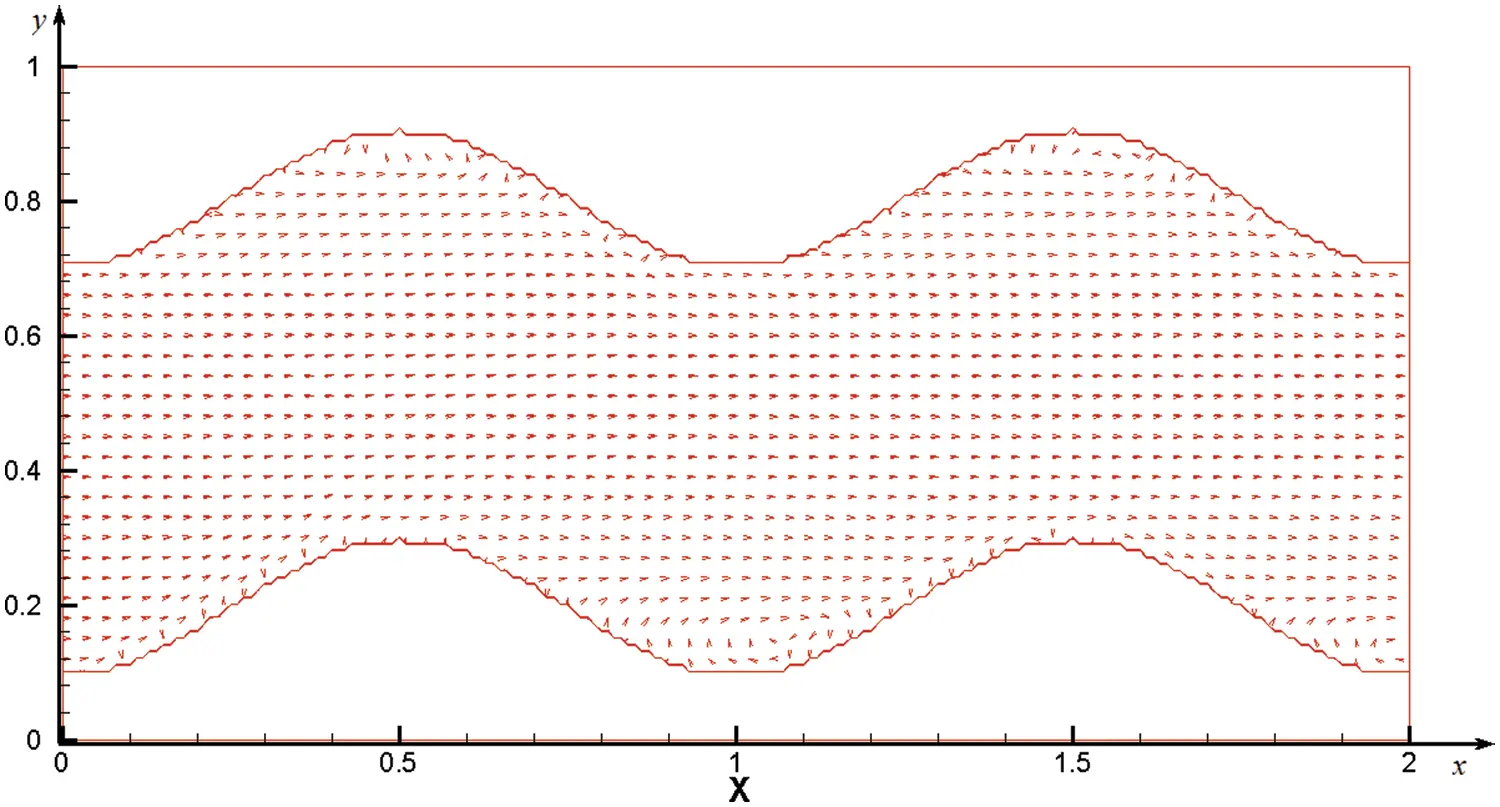Figure 3: Vector field of the problem solved using the fictitious domain method with the grid size 200×80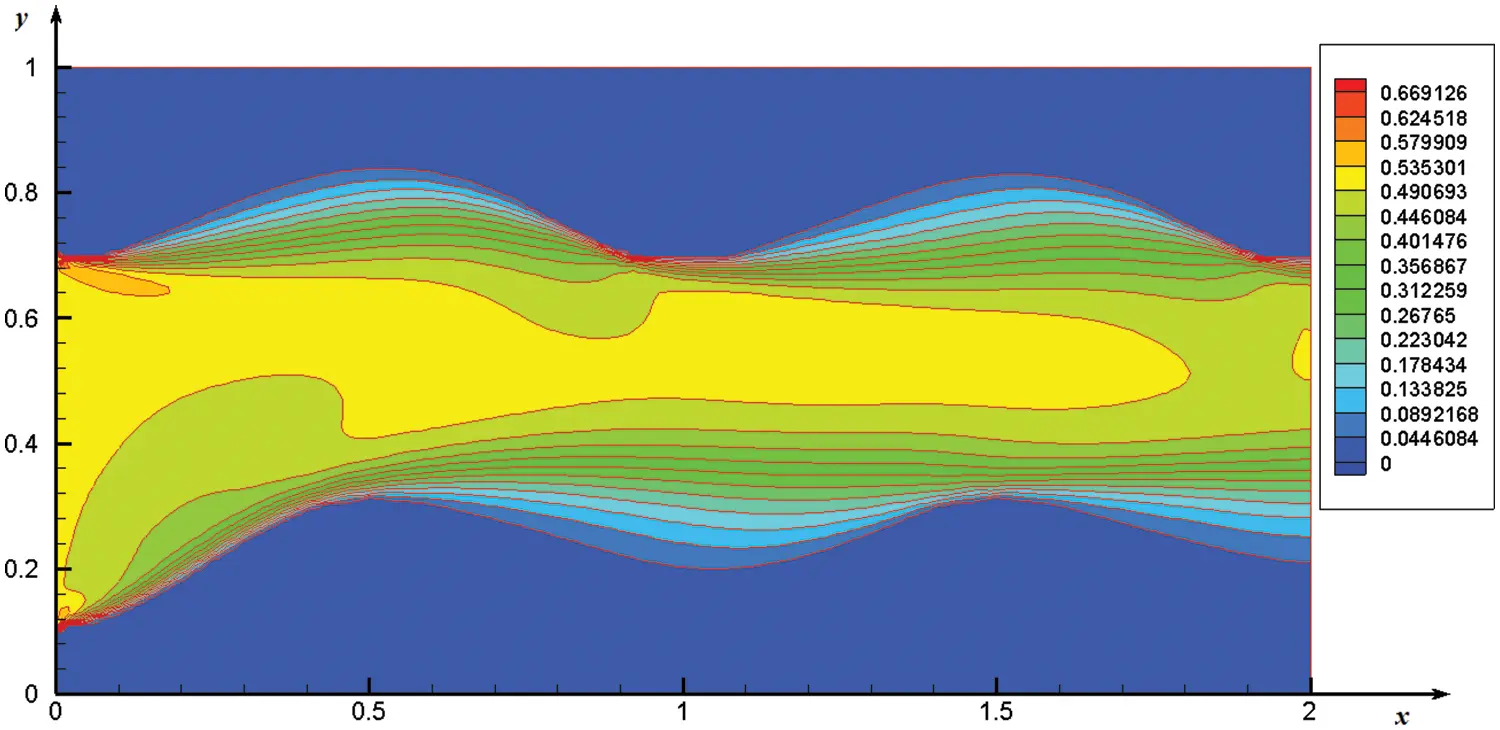Figure 4: The speed module of the problem solved using the fictitious domain method, grid size 200×80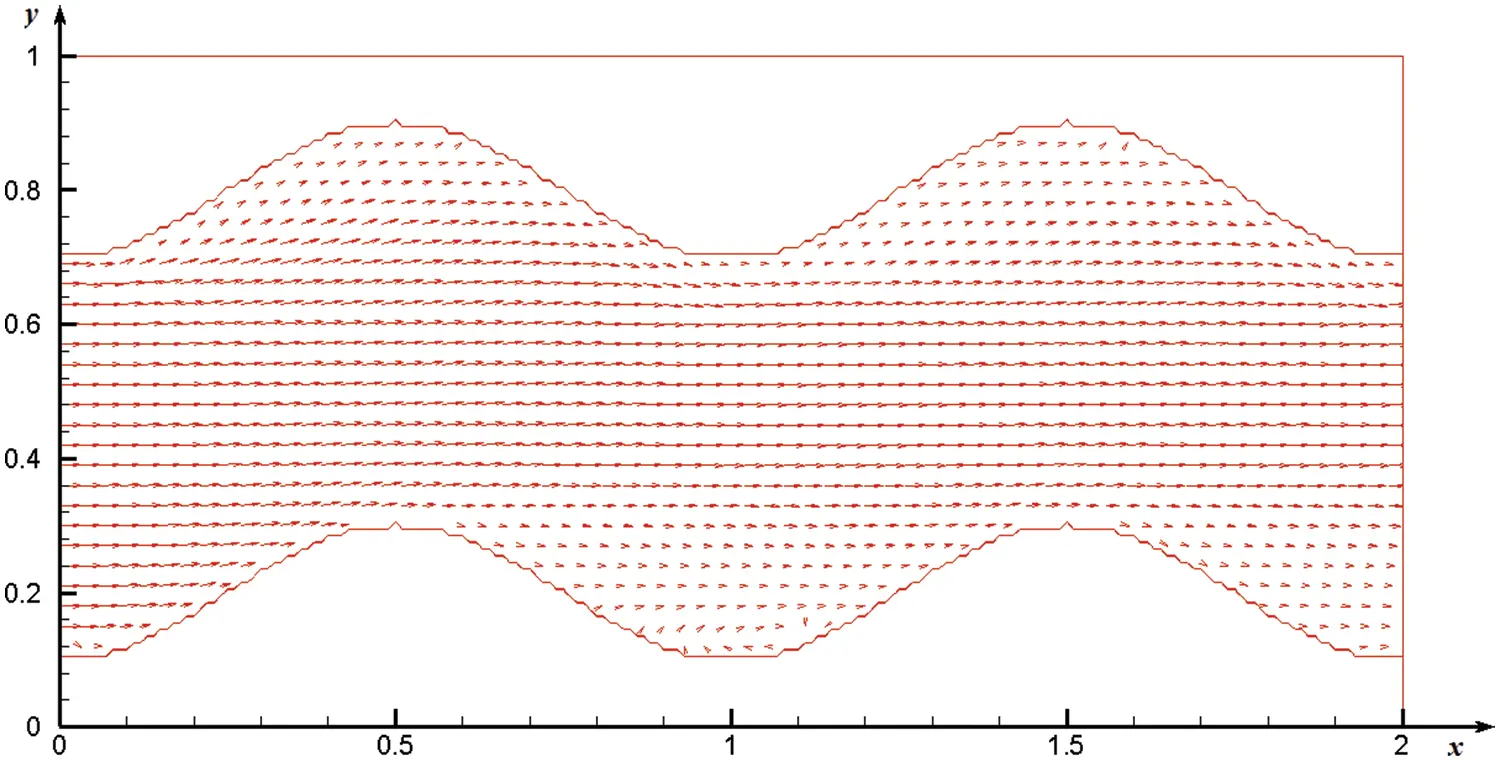Figure 5: Vector field of the problem solved on a consistent grid with the grid size 200×80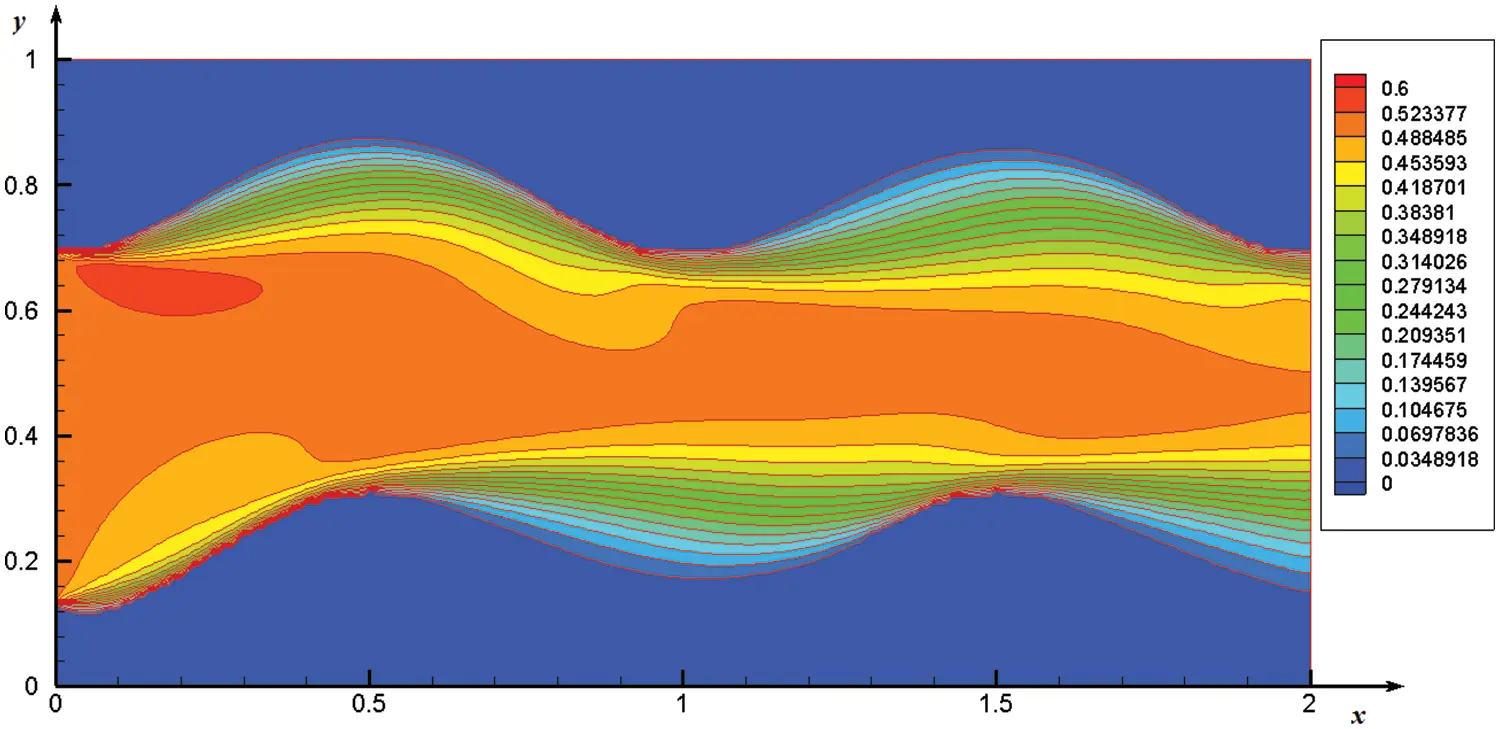Figure 6: The speed module of the problem solved on a consistent grid, the grid size is 200×80

Tab. 2 shows the maximum values of the velocity components on the indicated sections of the channel and their discrepancies. It follows from the table that there are small deviations which indicate a quantitative coincidence of the indicators.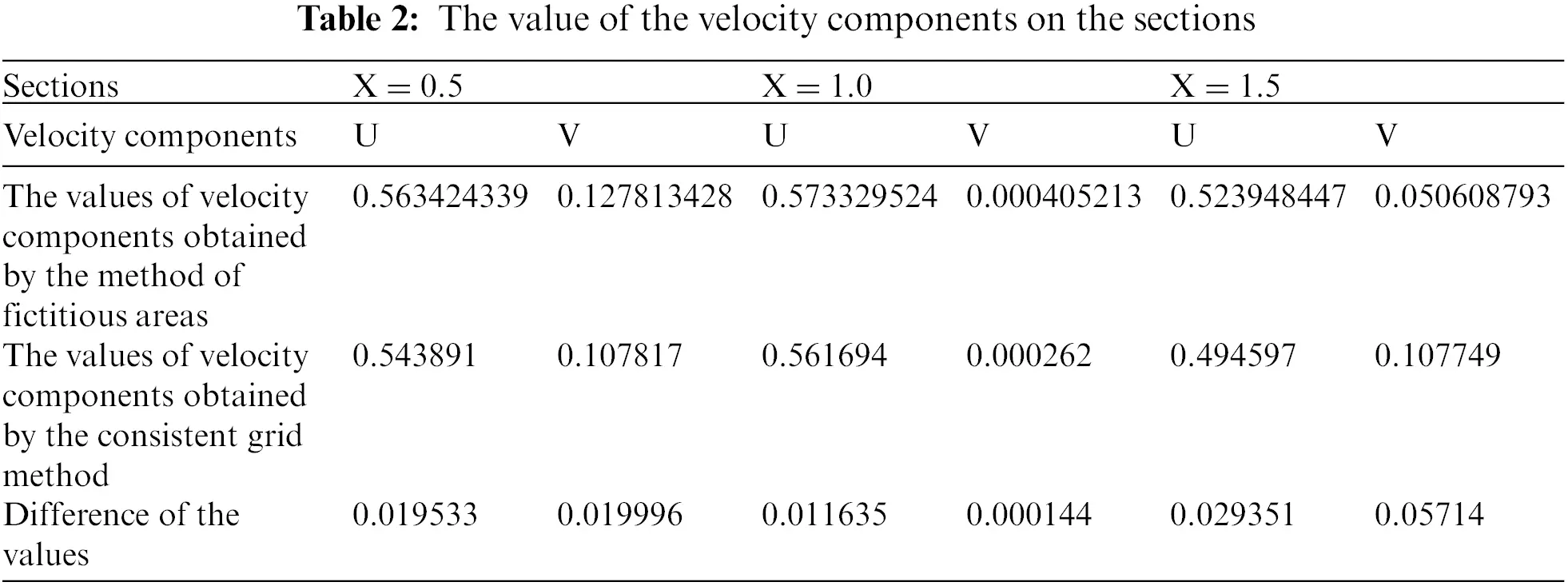It can be seen from Figs. 79 that the lower curved boundary relative to the computation domain is convex, and the upper one, on the contrary, is concave in the area of the section X = 0.5 and X = 1.5. This affects the profile values of the velocity component U at the lower boundary to a greater extent than at the upper boundary since they flow around the hill at the lower boundary and fall into the concavity pit at the upper boundary (Fig. 5). The values of the velocity component V are positive everywhere since the fluid flow moves in a positive direction(upward). Also, it can be seen that the values of the velocity component U2 have negative values in the border areas of the upper boundary which indicates the formation of vortex motions in the cavities.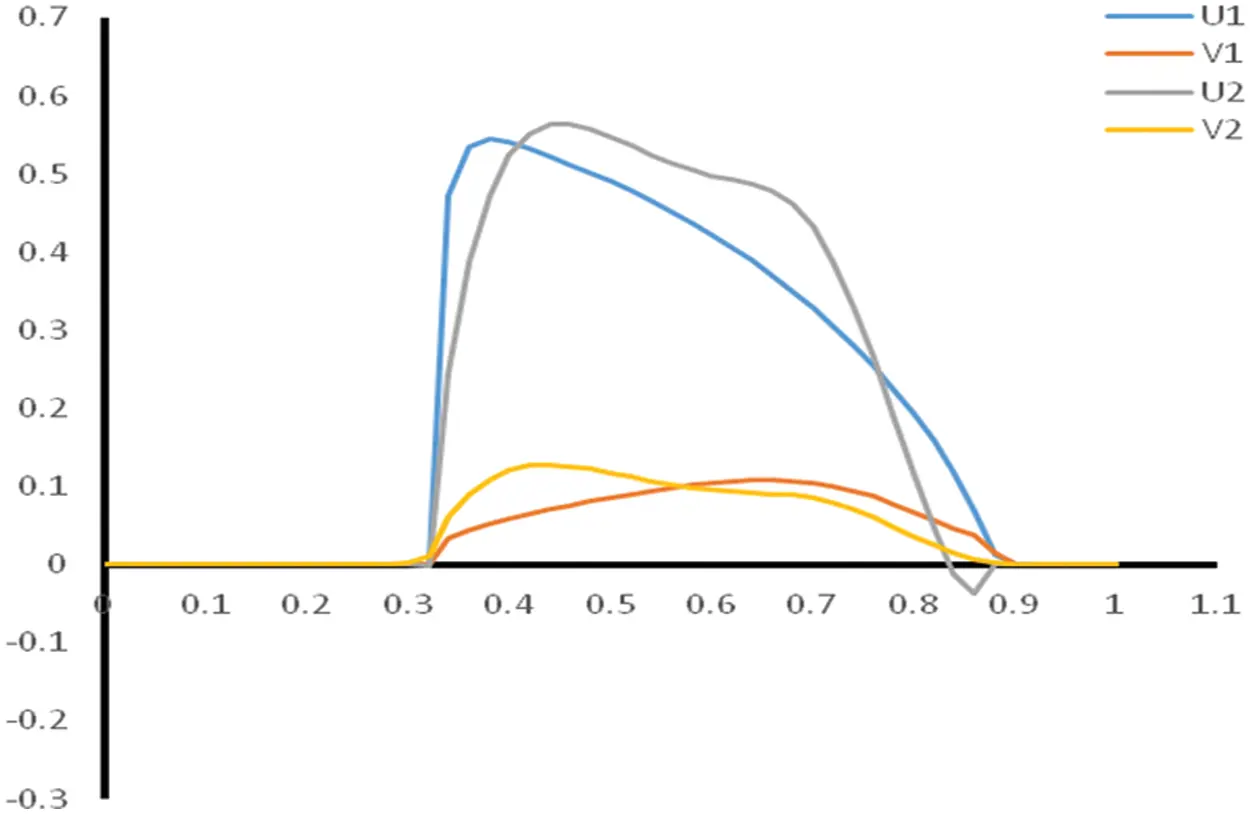Figure 7: Velocity profile U and V on the cross section at x =  0.5 (U1, V1 are the solution of the problem obtained on a consistent grid, U2, V2 are the solution of the problem obtained using the fictitious domains method)

In Fig. 8, on the contrary, the values of the velocity component U at the upper boundary are greater than at the lower one. Vortex motions are observed near the lower boundary from the presented graph of the velocity component U2. The values of the velocity component V are negative since the flow moves in a decreasing direction relative to the y axis(downward). The results presented in Fig. 7 are similar to those obtained in Fig. 5.Figure 8: Velocity profile U and V on the section at X =  1.0 (U1, V1 are the solution of the problem obtained on a consistent grid, U2, V2 are the solution of the problem obtained using the fictitious domains method)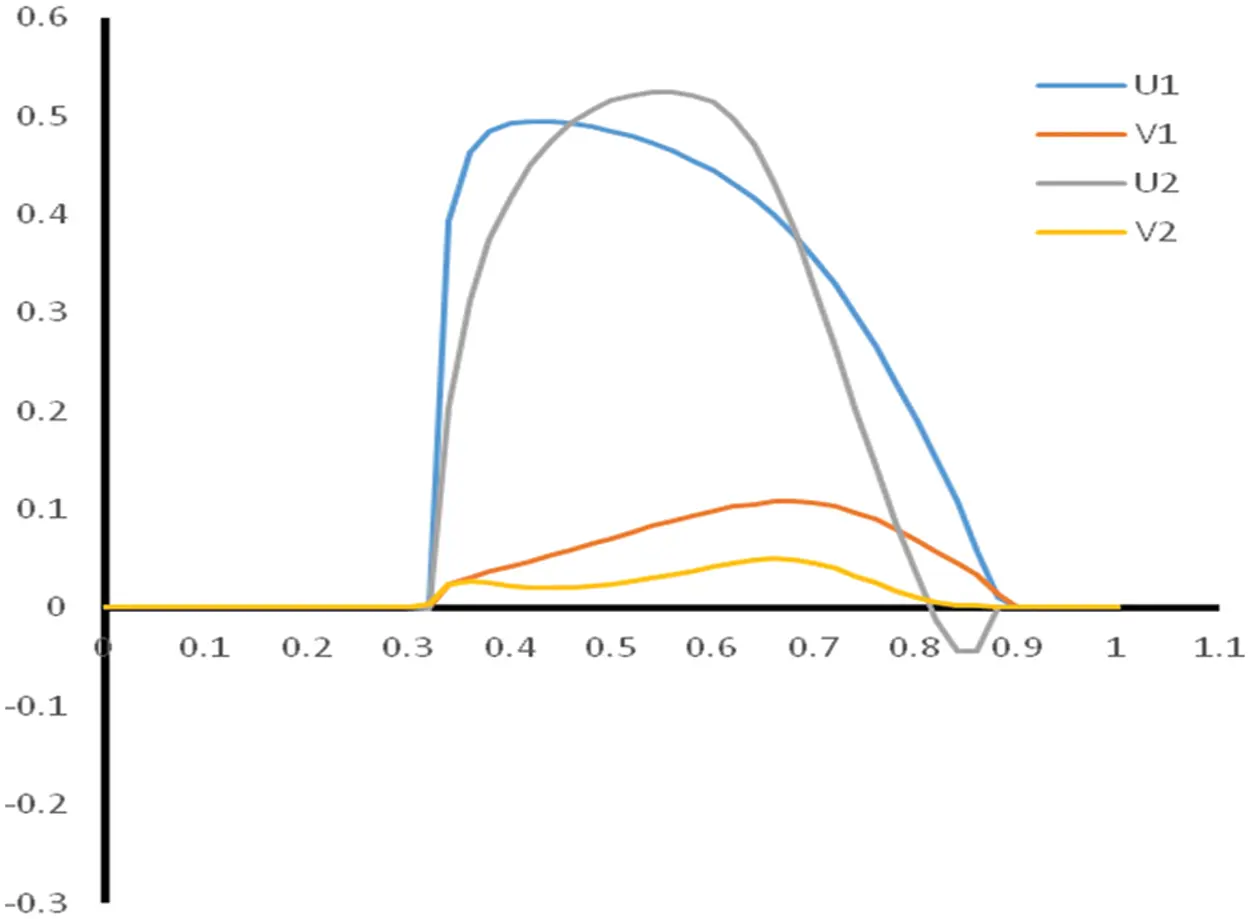Figure 9: Velocity profile U and V on the cross section at x =  1.5 (U1, V1 are the solution of the problem obtained on a consistent grid, U2, V2 are the solution of the problem obtained using the fictitious domains method)

The calculations used a uniform grid with dimensions of 50×20,100×40,150×60,200×80. A numerical experiment was carried out on a modern personal computer with the following characteristics: Intel(R) Core(TM)i9-10900F CPU@2.80GHz, RAM 32 GB.

Thus, in order to apply the fictitious domain method and conduct numerical experiments, the boundary value problem for an ordinary differential equation is first considered. The results of numerical calculations for different values of the iterative parameter τ and the small parameter ε are presented. After successful application of the fictitious domain method for an ordinary differential equation, a more complex problem of applying the fictitious domain method for the Navier-Stokes equation in natural variables is considered.

Further, the research of the auxiliary FDM problem at the differential level for the Navier-Stokes equations with continuation into a fictitious subdomain by the higher coefficients with a small parameter is carried out. Methods of a priori estimates are used for the mathematical study of the problems under consideration. A generalized solution of the auxiliary FDM problem with continuation by higher coefficients with a small parameter is determined. The fictitious domain method is used to solve many problems of computational fluid dynamics. Currently, there are several methods for the numerical solution of boundary value problems in complex geometric domains, such as the method of curved grids and fictitious domain method. The construction of curved grids for the numerical solution of problems requires the transformation of the equation into curved coordinates, which has a more complex form than the original equations. Therefore, for the numerical solution of a wide class of problems of mathematical physics in an arbitrary domain, it is effective to use the fictitious domain method.

Thus, in this paper, two methods are applied for the numerical solution of the formulated problem. The first one is the fictitious domain method associated with the modification of nonlinear terms in a fictitious subdomain. The model problem shows the efficiency of using such a modification. The proposed variation of the method is used to solve two problems at once that arise in the numerical solution of the Navier-Stokes equations: the problem of the curvilinear boundary of an arbitrary domain and the problem of the absence of a boundary condition for pressure in the physical formulation of the internal flow problem. The main advantage of this method is its versatility in the development of computer programs.

The second method used the calculation on a uniform mesh inside the domain. The solution on a uniform mesh inside the domain makes it possible to accurately take into account the boundaries of the curved domain and ensures the accuracy of the function value on the boundaries of the domain in the numerical implementation. Tabs. 3 and 4 present the computation time and number of iterations obtained with the use of the two methods for different grid nodes. It can be seen from the presented tables that the calculation time and the number of iterations are noticeably longer in case of a numerical solution on a uniform grid inside the domain. This is due to the fact that the conditions for belonging of the calculation node to the computational area are checked at each stage of the algorithm. This affects the counting time and the number of iterations.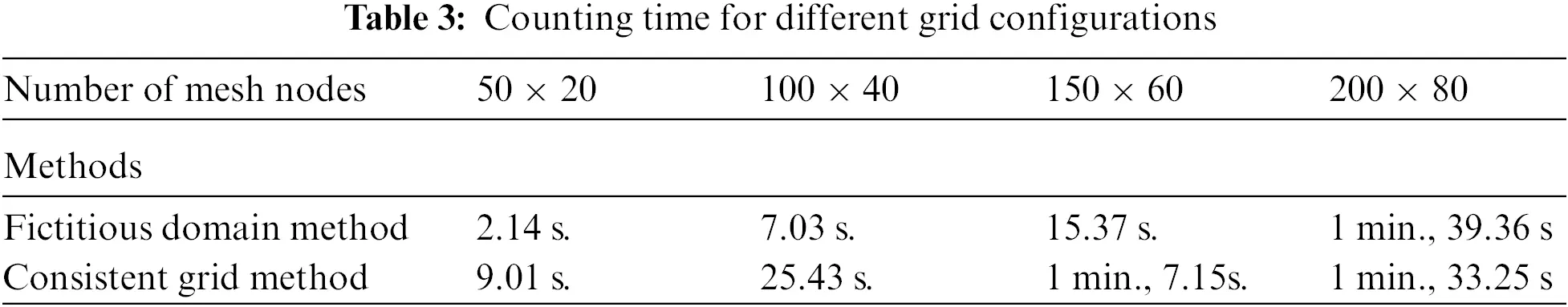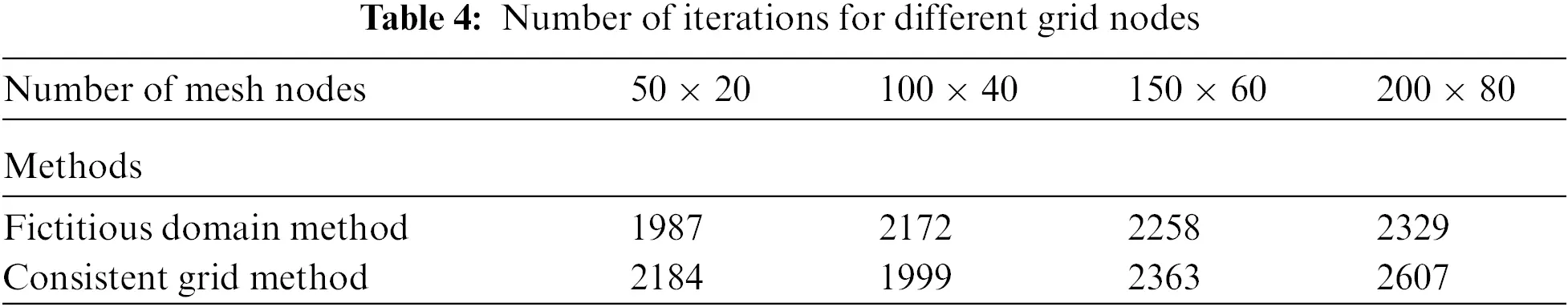Funding Statement: This research is funded by the Science Committee of the Ministry of Education and Science of the Republic of Kazakhstan (Grant No. AP09058430).

Conflicts of Interest: The authors declare that they have no conflicts of interest to report regarding the present study.

## References

1. P. N. Vabishevich, Method of Fictitious Domains for the Problem of Mathematical Physics, Moscow: Publishing House of Moscow State University, pp. 156, 199
2. N. A. Konovalov, “Method of fictitious domains,”. in Problems of Multiphase Fluid Filtration, 1st ed., Singapore: World Scientific, pp. 38–57, Chapter 5, 1994
3. R. Glowinski and T. W. Pan, “Error estimates for fictitious domain/penalty/finite element methods,” Calcolo, vol. 29, no. 1-2, pp. 125–141, 1992.
4. R. Glowinski and Y. A. Kuznetsov, “Distributed Lagrange multipliers based on fictitious domain method for second order elliptic problems,” Computer Methods in Applied Mechanics and Engineering, vol. 196, no. 8, pp. 1498–1506, 2007.
5. R. Glowinski, T. Pan and J. Periaux, “A fictitious domain method for external incompressible flow modeled by Navier-Stokes equations,” Computer Methods in Applied Mechanics and Enginering, vol. 112, no. 1-4, pp. 133–148, 1994.
6.    R. Glowinski, T. Pan, T. I. Hesla, D. D. Joseph and J. Periaux, “A distributed Lagrange multiplier/fictitious domain method for the simulation of flow around moving rigid bodies: Application to particulate flow,” Computer Methods in Applied Mechanics and Engineering, vol. 184, no. 2–4, pp. 241–267, 2000.
7.    V. Girault, R. Glowinski, H. López and J. P. Vila, “A boundary multiplier/fictitious domain method for the steady incompressible Navier-Stokes equations,” Numerische Mathematik, vol. 88, no. 1, pp. 75–103, 2001.
8. R. Glowinski, T. Pan, T. I. Hesla, D. D. Joseph and J. Périaux, “A fictitious domain approach to the direct numerical simulation of incompressible viscous flow past moving rigid bodies: Application to particulate flow,” Journal of Computational Physics, vol. 169, no. 2, pp. 363–426, 2001.
9. A. N. Bugrov and Sh. S. Smagulov, “The method of fictitious regions in boundary value problems for the Navier-Stokes equation,” in Mathematical Models of Fluid Flow USSR Academy of Sciences Siberian Branch Institute of Theoretical and Applied Mechanics, Novosibirsk, pp. 79–89, 1978.
10. Sh. S. Smagulov and M. K. Orunkhanov, “Approximate method for solving the equation of hydrodynamics in multi-connected regions,” Reports Academy of Sciences of the USSR, vol. 260, no. 5, pp. 1078–1082, 1981.
11. Sh. S. Smagulov, N. T. Danaev and N. M. Temirbekov, “Modeling of boundary conditions for pressure and full head in hydrodynamic problems using the method of fictitious regions,” Reports Academy of Sciences of the Russian, vol. 374, no. 3, pp. 333–335, 2000.
12. M. Wu, B. Peters, T. Rosemann and H. Kruggel-Emden, “A forcing fictitious domain method to simulate fluid-particleinteraction of particles with super-quadric shape,” Powder Technology, vol. 360, no. 2, pp. 264–277, 2020.
13. Q. He, J. Huang, X. Shi, X. Wang and C. Bi, “Numerical simulation of 2D unsteady shear-thinning non-Newtonian incompressible fluid in screw extruder with fictitious domain method,” Computer and Mathematics with Applications, vol. 73, no. 1, pp. 109–121, 2017.
14. S. Court, “A fictitious domain approach for a mixed finite element method solving the two-phase Stokes problem with surface tension forces,” Journal of Computational and Applied Mathematics, vol. 359, no. 1, pp. 30–54, 2019.
15. Y. Wang, P. Jimack and M. Walkley, “A one-field monolithic fictitious domain method for fluid-structure interactions,” Computer Methods in Applied Mechanics and Engineering, vol. 317, no. 2, pp. 1146–1168, 2017.
16. M. Fournie and J. Morrison, “Fictitious domain for stabilization of fluid-structure interaction,” IFAC PapersOnLine, vol. 50, no. 1, pp. 12301–12306, 2017.
17. Y. Wang, P. Jimack and M. Walkley, “Energy analysis for the one-field fictitious domain method for fluid-structure interactions,” Applied Numerical Mathematics, vol. 140, no. 5, pp. 165–182, 2019.
18. D. Wacks, M. E. Nakhchi and M. Rahmati, “Forced response of a low-pressure turbine blade using spectral/HP element method: Direct numerical simulation,” Applied and Computational Mechanics, vol. 7, pp. 135–147, 2021.
19. Q. He, R. Glowinski and X. Wang, “A least-squares/fictitious domain method for incompressible viscous flow around obstacleswith navier slip boundary condition,” Journal of Computational Physics, vol. 366, no. 3, pp. 281–297, 2018.
20. G. Zhou, “The fictitious domain method with H1-penalty for the Stokes problem with dirichlet boundary condition,” Applied Numerical Mathematics, vol. 123, no. 3–4, pp. 1–21, 2018.
21. H. R. Mottahedi, M. Anbarsooz and M. Passandideh-Fard, “Application of a fictitious domain method in numerical simulation of an oscillating wave surge converter,” Renewable Energy, vol. 121, no. 5459, pp. 133–145, 2018.
22. C. Wang and P. Sun, “A fictitious domain method with distributed lagrange multiplier for parabolic problems with moving interfaces,” Journal of Scientific Computing, vol. 70, no. 2, pp. 686–716, 2017.
23. Kh. Lotfy, A. A. El-Bary, W. Hassan, A. R. Alharbi and M. B. Almatrafi, “Electromagnetic and Thomson effects during photothermal transport process of a rotator semiconductor medium under hydrostatic initial stress,” Results in Physics, vol. 16, pp. 1–9, 2020.
24. P. Sun and C. Wang, “Distributed lagrange multiplier/fictitious domain finite element method for Stokes/parabolic interface problems with jump coefficients,” Applied Numerical Mathematics, vol. 152, no. 4, pp. 199–220, 2020.
25. P. Sun, “Fictitious domain finite element method for Stokes/elliptic interface problems with jump coefficients,” Journal of Computational and Applied Mathematics, vol. 356, no. 3, pp. 81–97, 2019.
26. A. N. Temirbekov and W. Wojcik, “Numerical implementation of the fictitious domain method for elliptic equations,” International Journal of Electronics and Telecommunications, vol. 60, no. 3, pp. 219–223, 2014.
27. A. N. Temirbekov, “Numerical implementation of the method of fictitious domains for elliptic equations,” in Int. Conf. on Analysis and Applied Mathematics (ICAAM 2016). AIP Conf. Proc., Almaty City, Kazakhstan, pp. 020053-1–020053-6, 2016.
28. A. R. Alharbi, M. B. Almatrafi and Kh. Lotfy, “Constructions of solitary travelling wave solutions for Ito integro-differential equation arising in plasma physics,” Results in Physics, vol. 19, no. 1, pp. 103533, 2020.
29. M. B. Almatrafi, A. R. Alharbi, Kh. Lotfy and A. A. El-Bary, “Exact and numerical solutions for the GBBM equation using an adaptive moving mesh method,” Alexandria Engineering Journal, Novosibirsk, vol. 60, no. 5, pp. 4441–4450, 2021.
30. E. Heikkola, T. Rossi and J. Toivanen, “A parallel fictitious domain method for the three-dimensional helmholtz equation,” SIAM Journal on Scientific Computing, vol. 24, no. 5, pp. 1567–1588, 2000.
31. Z. Yu, Z. Lin, X. Shao and L. Wang, “A parallel fictitious domain method for the interface-resolved simulation of particle-laden flows and its application to the turbulent channel flow,” Engineering Applications of Computational Fluid Mechanics, vol. 10, no. 1, pp. 160–170, 2016.
32. M. Ruess, V. Varduhn, E. Rank and Z. Yosibash, “A parallel high-order fictitious domain approach for biomechanical applications,” in 2012 11th Int. Symp. on Parallel and Distributed Computing, Munich, Germany, pp. 279–285, 2012.
33. Kh. Lotfy and M. Othman, “Effect of rotation on plane waves in generalized thermo-microstretch elastic solid with a relaxation time,” Meccanica, vol. 47, no. 6, pp. 1467–1486, 2012.
34. Kh. Lotfy, “Effect of variable thermal conductivity during the photothermal diffusion process of semiconductor medium,” Silicon, vol. 11, no. 4, pp. 1863–1873, 2019.
35. A. Temirbekov, D. Baigereyev, N. Temirbekov, B. Urmashev and A. Amantayeva, “Parallel CUDA implementation of a numerical algorithm for solving the Navier-Stokes equations using the pressure uniqueness condition,” in Int. Conf. on Analysis and Applied Mathematics (ICAAM 2020). AIP Conf. Proc., Mersin, Turkey, pp. 20063, 2021.
36. N. Temirbekov, S. Tokanova and Y. Malgazhdarov, “Information technology for numerical simulation of viscous incompressible flow in biconnected domains,” Journal of Theoretical and Applied Information Technology, vol. 88, pp. 441–448, 2016.
37. R. Glowinski, T. Pan and J. Periaux, “A fictitious domain method for dirichlet problem and applications,” Computer Methods in Applied Mechanics and Enginering, vol. 111, no. 3-4, pp. 283–303, 1994.
38. R. Glowinski, T. Pan and J. Periaux, “A Lagrange multiplier/fictitious domain method for the dirichlet problem. generalization to some flow problems,” Japan Journal of Industrial and Applied Mathematics, vol. 12, no. 1, pp. 87–108, 1995.
39. R. Glowinski, T. Pan, A. Kearsley and J. Periaux, “Numerical simulation and optimal shape for viscous flow by a fictitious domain method,” International Journal for Numerical Methods in Fluids, vol. 20, pp. 695–711, 1995.
40. A. A. Ivanchikov, “Numerical solution of some spectral problems for Stokes equations,” Computational Methods and Programming, vol. 4, no. 1, pp. 227–243, 2003.
41. S. Gallier, E. Lemaire, L. Lobry and F. Peters, “A fictitious domain approach for the simulation of dense suspensions,” Journal of Computational Physics, Elsevier, vol. 256, pp. 367–387, 2014.
42. O. M. Belotserkovsky, V. A. Gushchin and V. V. Schennikov, “Splitting method applied to solving problems of dynamics of a viscous incompressible fluid,” Computational Mathematics and Mathematical Physics, vol. 15, no. 1, pp. 197–207, 1975.
43. P. Roach, “Basic numerical methods for calculating incompressible fluid motions,” in Computational fluid dynamics, Moscow, Russia: Mir, Chapter 3, pp. 117–138, Mir, Chapter 3, 1980.
44. K. M. Khazimov, G. C. Bora, B. A. Urmashev, M. Z. Khazimov and M. Z. Khazimov, “Computation of optimal structural and technical parameters of solar dryer,” International Journal of Engineering and Innovative Technology (IJEIT), vol. 4, pp. 251–261, 2014.This work is licensed under a Creative Commons Attribution 4.0 International License, which permits unrestricted use, distribution, and reproduction in any medium, provided the original work is properly cited.